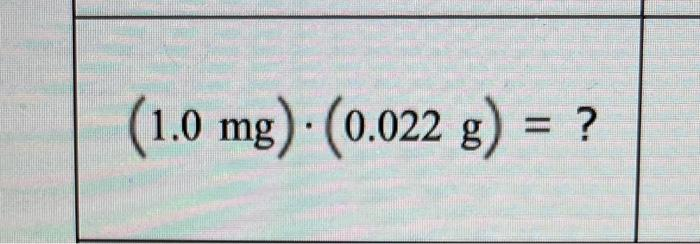decide whether each proposed multiplication or division of measurements is possible.
(1.0mg)x(0.022g) =?$$(1.0 \mathrm{mg}) \cdot(0.022 \mathrm{~g})=?$$

We have an Answer from Expert